# Thevenin Equivalent Circuit

## Thevenin Equivalent Circuit:

In many practical applications, it is always not necessary to analyse the complete circuit; it requires that the voltage, current, or power in only one resistance of a circuit be found. The use of this theorem provides a simple, equivalent circuit which can be substituted for the original network. Thevenin Equivalent Circuit states that any two terminal linear network having a number of voltage current sources and resistances can be replaced by a simple equivalent circuit consisting of a single voltage source in series with a resistance, where the value of the voltage source is equal to the open circuit voltage across the two terminals of the network, and resistance is equal to the equivalent resistance measured between the terminals with all the energy sources are replaced by their internal resistances. According to Thevenin’s theorem, an equivalent circuit can be found to replace the circuit in Fig. 3.13.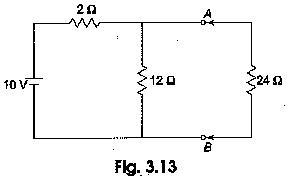In the circuit, if the load resistance 24 Ω is connected to Thevenin’s equivalent circuit, it will have the same current through it and the same voltage across its terminals as it experienced in the original circuit. To verify this, let us find the current passing through the 24 Ω resistance due to the original circuit.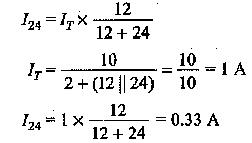The voltage across the 24 Ω resistor = 0.33 x 24 = 7.92 V. Now let us find Thevenin Equivalent Circuit.

The Thevenin voltage is equal to the open circuit voltage across the terminals ‘AB’, i.e. the voltage across the 12 Ω resistor. When the load resistance is disconnected from the circuit, the Thevenin voltage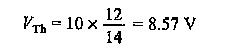The resistance into the open circuit terminals is equal to the Thevenin resistance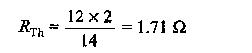Thevenin Equivalent Circuit is shown in Fig. 3.14.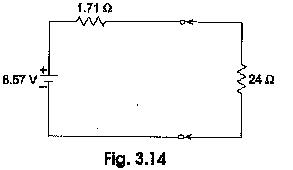Now let us find the current passing through the 24 Ω resistance and voltage across it due to Thevenin Equivalent Circuit.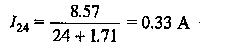The voltage across the 24 Ω resistance is equal to 7.92 V. Thus, it is proved that RL (= 24 Ω) has the same values of current and voltage in both the original circuit and Thevenin Equivalent Circuit.